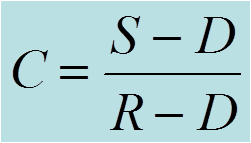# Useful Formulas

### Microscopy Formulas

Definitions: ‘n’  is the refractive index,  λ’ is the wavelength,  ‘NA’ is the Numerical Aperture and ‘f’ is the focal length

Resolving Power: Also called spatial resolution or horizontal resolution, is the minimum distance between two points that may be resolved by the microscope.  The resolution formula of a microscope is:Depth of Field: This is the longitudinal resolving power of the microscope.  It is the distance between the nearest object plane that is in focus and the farthest object plane that is also in focus.  It is defined by the equation: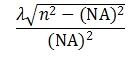Useful magnification Range for an optical microscope at 550 nm ranges from a minimum of [500 X (NA of the objective)] to a maximum of
[1000 X (NA of the objective)]

The Refractive Index (n) is ratio of the speed of light in a lower density media (such as air or oil) divided by the speed of light in a higher density media (such as quartz).

The Working Distance (WD) of a microscope objective is the distance from the front lens element of the objective to the surface of the sample when it is in focus.

Parfocal objectives stay in focus on an object when changed between one objective and another with only a small variation in focus.

Parcentral objectives have the same centering on the object when changing between one objective and another with only a small amount of error.

Some microscopy formulas can also be related to standard optical terminology:The Numerical Aperture is defined as the ability of a lens, or grouping of lenses such as a microscope objective, to collect light and resolve image details at a fixed object distance.  The numerical aperture formula defines it as: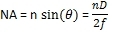The f-number of a microscope is the working distance of the objective divided by the aperture diameter.  It is defined by the equation: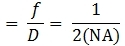### Spectroscopy Formulas

Einstein-Planck equation shows that light energy is related to its wavelength.  The equation is:Transmittance is defined by intensity of radiant energy transmitted through the object divided by the intensity of radiant energy illuminating the object at each wavelength.

Absorbance is related to the transmittance by the equation A = - log T.

The Beer Lambert equation states that the absorbance is equal to the molar absorptivity times the object thickness (b) times the absorber concentration (c).  The equation is: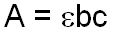Relative reflectance is defined by intensity of radiant energy reflected from the object divided by the intensity of radiant energy illuminating the object at each wavelength.  Please note that absolute reflectance adds additional terms to the equation to eliminate the spectral characteristics of the reference material.

Spectral Resolution is defined as the ability to resolve spectral features.  This is calculated by measuring the width of a narrow spectral peak at the half the peak maximum in terms of the wavelength.  This is also call the Full Width Half Maximum (FWHM).

Spectral Range is the region in which the spectrometer is sensitive.  For example, a spectrophotometer covering the visible range would have a spectral range of at least 400 to 700 nm.

Step Size is the spectral range divided by the number of pixels in the detector.  This is not related to the spectral resolution as the latter is also related to the entrance slit width and the spectrometer design.

Dark Scan (D) is acquired by measuring a spectrum with all light blocked from the detector.  This measures the electronic noise of the detector and associated electronics.

Reference Scan (R) is acquired by measuring a spectrum of a reference material or area on a sample.  This measures the spectral characteristics of the material as well as the optics, light sources and detectors of the system.

Sample Scan (S) is acquired by measuring a spectrum of the sample.  The following calculation is performed to generate a corrected spectrum of just the sample without any of the spectral characteristics of the system.